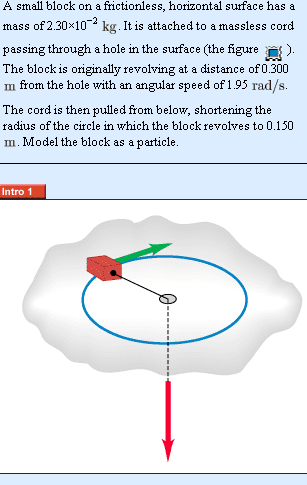# Conservation of Angular momentum

## Homework StatementWhy is angular momentum conserved?

## Homework Equations

$$\vec{\tau}= \vec{r} x \vec{F}$$
magnitude of $$\tau = I \alpha$$

## The Attempt at a Solution

I first don't agree on why angular momentum is conserved, because if the radius change doesn't that change the torque?

And by definition angular momentum of a system is conserved when there are no external torque. While for particles, angular moment is conserved when there is no change in torque.

So the torque changes when radius is shortened, why is it that angular momentum is conserved?

Last edited: